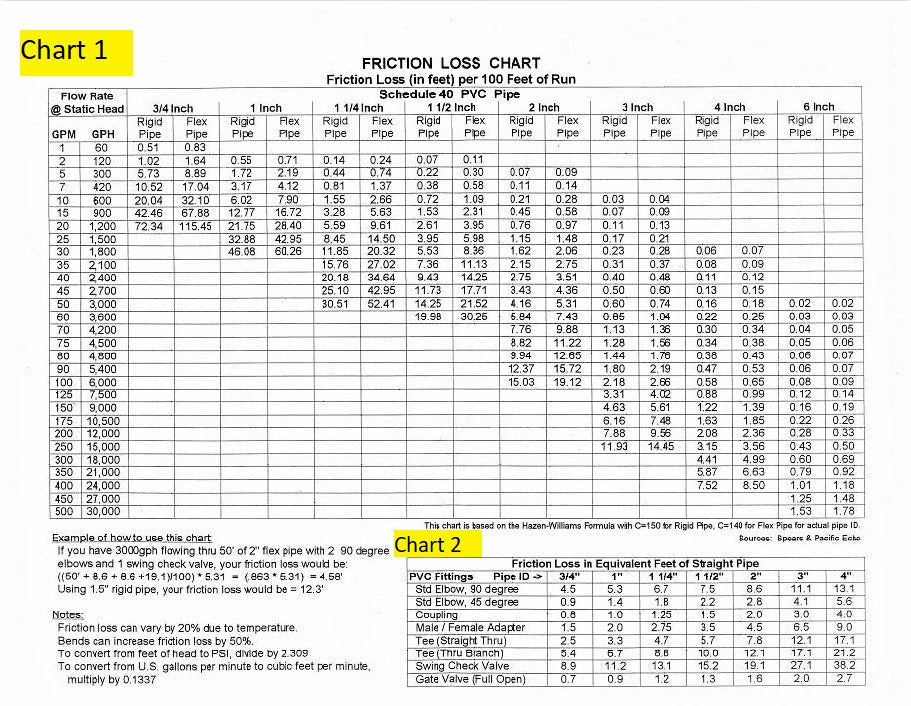# Pump Sizing for Pondless Systems

Step 1:  Stream Volume & Basin Volume

Before sizing a pump for your pondless system, you need to know the volume of water that your basin will need to hold.  You will need to calculate the volume of your stream.

The manual calculation for your stream volume is:

#### * Measurements are in feet and this is an average stream depth of 1 inch.

Your pump needs to be fully submerged while running and while the stream is full.  Aquascape recommends the basin to be twice the volume of your stream volume.

#### Stream Volume x 2 = Gallons in Basin

You can also use the calculator below:

Step 2:  Flow Rate

It is recommended between 100 - 200 gallons per hour (GPH) for every inch of waterfall width.  On the lower flow rate (100 GPH) will give you a slower stream vs the higher flow rate (200 GPH) will give you a faster stream over your waterfall.  Aquascape recommends 125 GPH for every inch of waterfall.  Use this calculator to help you calculate your low and high flow rates.#### Waterfall Width x 125 = Approximate Flow Rate*

*Waterfall width measured in inches

Elevation (Static Head):  Height difference from water level in the feature to the top of the waterfall.

Pipe Length:  Total length of pipe (10 feet of pipe = 1 foot of head height)

Fittings Used (For simple ponds, this can be ignored): 90-degree fittings (equate to 2 feet of head height) and all other fittings (1 foot of head height) contribute to head losses.   However, on larger applications you also need to factor in friction loss from the pipe as well as each fitting to get the Total Dynamic Head (TDH), shown in Step 4.

Use the calculator below to calculate Static Head.Step 5. Calculate Total Dynamic Head for Larger Applications

Total Dynamic Head is the Static Head, found in Step 3 + the friction loss created by the pipe and the fittings.  In most small ponds with short pipe runs friction loss is not typically a problem.  However, in a with long streams or high flow rates, friction loss can have a big impact on the performance of the pump.

Use our calculator below to find your Total Dynamic Head.   Friction Loss numbers are available on Chart 1 & Chart 2 below and are based on your diameter of pipe and whether it is flexible PVC or rigid PVC.

Here's an example on obtaining friction loss numbers: We are pumping 4200 GPH through 100' of 2" flex pipe.  We are also using 2 - 2" 90-degree elbows and 1 - 2" check valve.

Friction Loss for the 2" Flex Pipe:  Using Chart 1, the pipe friction loss for the 2" pipe is 9.98.  Follow the 4,200 GPH in the second column from the left over to the 2-inch Flex Pipe column.

Friction Loss for the 2" 90-Degree Elbows:  Using Chart 2, the pipe friction loss for the 2" - 90-Degree Elbows is 8.6 for EACH elbow.  Follow the "Std Elbow, 90 Degree" in the left column over to the "2" " column.

Friction Loss for the 2" Check Valve:  Using Chart 2, the pipe friction loss for the 2" - Check Valve is 19.1.  Follow the "Swing Check Valve" in the left column over to the "2" " column.

These friction loss numbers shown in the above example can be added to the Total Dynamic Head Calculator below.